Q-Chem 5.1 User’s Manual

# 5.12 Thermally-Assisted-Occupation Density Functional Theory (TAO-DFT)

Aiming to study the ground-state properties of large, strongly correlated systems with minimum computational complexity, Prof. Jeng-Da Chai recently developed thermally-assisted-occupation density functional theory (TAO-DFT). Unlike conventional multi-reference methods, the computational complexity of TAO-DFT increases very insignificantly with the size of the active space (i.e., an active space restriction is not needed for TAO-DFT calculations), and TAO-DFT appears to be very promising for the study of large poly-radical systems. TAO-DFT is a DFT scheme with fractional orbital occupations produced by the Fermi-Dirac distribution, controlled by a fictitious temperature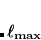, and existing XC functionals (e.g., LDA or GGAs) can be used in TAO-DFT. The computational cost of the method is similar to that of KS-DFT for single-point energy calculations and analytical nuclear gradients, and reduces to the cost of KS-DFT in the absence of strong static correlation effects.

There are several $rem variables that are used for TAO-DFT. TAO_DFT  Controls whether to use TAO-DFT. TYPE:  Boolean DEFAULT:  false OPTIONS:  false Do not use TAO-DFT true Use TAO-DFT RECOMMENDATION:  NONE TAO_DFT_THETA  Controls the value of the fictitious temperaturein TAO-DFT. TYPE:  INTEGER DEFAULT:  7 OPTIONS: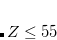(hartrees), where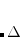is the value of TAO_DFT_THETA_NDP RECOMMENDATION:  NONE TAO_DFT_THETA_NDP  Controls the value of the fictitious temperaturein TAO-DFT. TYPE:  INTEGER DEFAULT:  3 OPTIONS:(hartrees), whereis the value of TAO_DFT_THETA RECOMMENDATION:  NONE Note that setting TAO_DFT_THETA = 0 recovers ordinary KS-DFT. In addition to the XC functional, a functional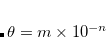is needed in TAO-DFT. Currently available in Q-Chem are an LDA version (the ETheta_LDA functional) as well as a version based on the gradient expansion approximation (GEA) (ETheta_GEA functional), and the latter may be substituted for the former in the sample jobs below. Example 5.63 TAO-LDA calculation on Be atom $molecule
0 1
Be
$end$rem
JOBTYPE             sp
BASIS               6-31G*
EXCHANGE            gen
TAO_DFT             true
TAO_DFT_THETA       7    ! default, theta=7 mhartree
TAO_DFT_THETA_NDP   3    ! default
$end$xc_functional
X   S             1.0
C   PW92          1.0
X   ETheta_LDA    1.0
$end  Example 5.64 TAO-PBE, spin-restricted calculation on stretched N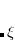$molecule
0 1
N1
N2 N1 4.5
$end$rem
JOBTYPE             sp
BASIS               6-31G*
EXCHANGE            gen
TAO_DFT             true
TAO_DFT_THETA       40  ! theta = 40 mhartree
TAO_DFT_THETA_NDP   3
$end$xc_functional
X PBE         1.0
C PBE         1.0
X ETheta_LDA  1.0
$end  Example 5.65 TAO-PBE, spin-unrestricted calculation on stretched N$molecule
0 1
N1
N2 N1 5.0
$end$rem
JOBTYPE             opt
UNRESTRICTED        true
BASIS               6-31G*
EXCHANGE            gen
TAO_DFT             true
TAO_DFT_THETA       40  ! theta = 40 mhartrees
TAO_DFT_THETA_NDP   3   ! can omit this line
SCF_GUESS           gwh
SCF_GUESS_MIX       3   ! mix in 30% LUMO in alpha to break symmetry
GEN_SCFMAN          FALSE
$end$xc_functional
X PBE         1.0
C PBE         1.0
X ETheta_LDA  1.0
\$end